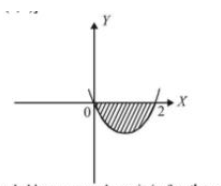Question

# A curve ′C′ passes through (2,0) and the slope at (x,y) is (x+1)2+(y−3)x+1. The area(in sq.units) bounded by curve and x−axis in fourth quadrant is:

A
23
No worries! We‘ve got your back. Try BYJU‘S free classes today!
B
43
Right on! Give the BNAT exam to get a 100% scholarship for BYJUS courses
C
83
No worries! We‘ve got your back. Try BYJU‘S free classes today!
D
13
No worries! We‘ve got your back. Try BYJU‘S free classes today!
Open in App
Solution

## The correct option is B 43 According to given data, slope of curve C at (x,y) is dydx=(x+1)2+(y−3)x+1 x≥0 in IVth quadrent.Now dydx=(x+1)+y−3x+1 ⇒dydx−(1x+1)y=x+1−3x+1 I.F.=e∫−1x+1dx=e−ln(x+1)=1x+1 ∴ Solution is y1x+1=∫[1−3(x+1)2]dx yx+1=x+3x+1+C y=x(x+1)+3+C(x+1) ⋯(1) As the curve passes throgh (2,0) ∴0=2⋅3+3+C⋅3 ⇒C=−3 ∴ Equation (1) becomes y=x(x+1)+3−3x−3 y=x2−2x ⋯(2) which is the required equation of curve. This can be written as (x−1)2=(y+1) It is an upward parabola with vertex at (1,−1) meeting x−axis at (0,0) and (2,0)Area bounded by curve and x−axis in fourth quadrant is as shown in the shaded region in figure and is given by: A=∣∣ ∣∣2∫0y dx∣∣ ∣∣=∣∣ ∣∣2∫0(x2−2x) dx∣∣ ∣∣=[x33−x2]20 ⇒A=∣∣∣83−4∣∣∣=43 sq. unitsSuggest Corrections0Similar questions
Join BYJU'S Learning Program
Select...Explore more
Join BYJU'S Learning Program
Select...